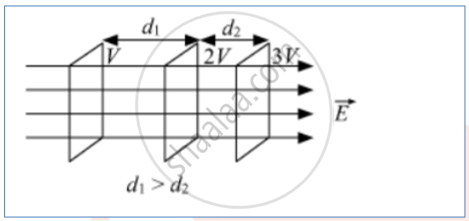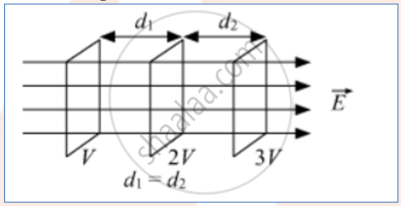# Draw Equipotential Surfaces Corresponding to a Uniform Electric Field in the Z-directions. - Physics

Diagram

Draw equipotential surfaces corresponding to a uniform electric field in the z-directions.

#### Solution

For constant electric field vector EFor increasing electric fieldDifference:

For constant electric field, the equipotential surfaces are equidistant for the same potential difference between these surfaces; while for increasing electric field, the separation between these surfaces decreases, in the direction of the increasing field, for the same potential difference between them.

Concept: Electric Field - Introduction of Electric Field
Is there an error in this question or solution?